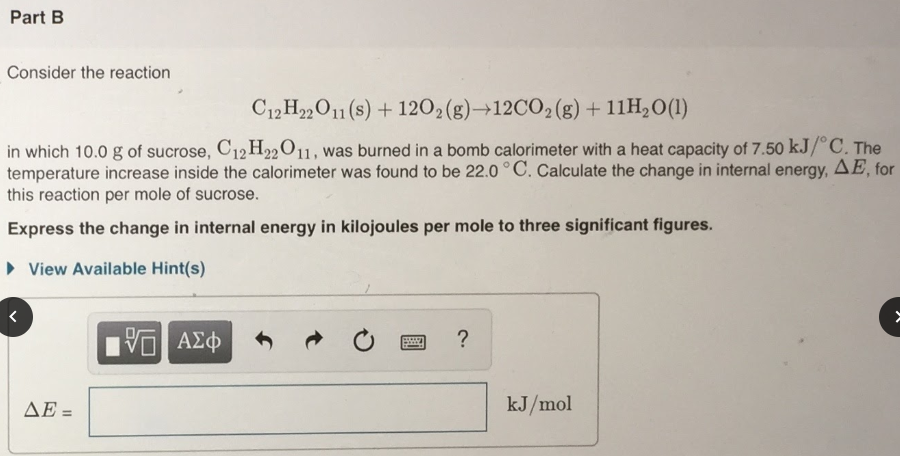# Consider the reaction C12H22O11(s) + 12O2(g) → 12CO2(g) + 11H2O(l) in which 10.0 g of sucrose, C12H22O11, was burned in a bomb calorimeter with a heat capacity of 7.50 kJ/degrees C. The temperature increase inside the calorimeter was found to be 22.0 degrees C. Calculate the change in internal energy, ΔE, for this reaction per mole of sucrose. Express the change in internal energy in kilojoules per mole to three significant figures.